# Check if the given array can represent Preorder Traversal of a Binary Search Tree

In this article, we are going to see if a given array can represent preorder traversal of a Binary Search Tree?
Submitted by Radib Kar, on October 28, 2020

Here, we are going to see given an array how can we check whether the given array can represent preorder traversal of a binary search tree or not.

For example,

Let's take two example

Where one is a valid preorder traversal and another is not

Preorder1:

This is a valid preorder traversal for a binary Search Tree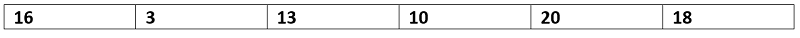Preorder2:

This is not a valid preorder traversal for a binary Search Tree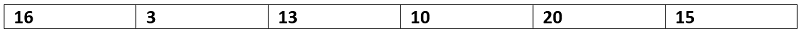Now, how we can figure out whether it's valid or not

In our article on constructing a BST from preorder traversal, we saw that the BST property helps us to distinguish b/w left subtree and the right subtree

If the array is of length n, then arr is the root

And say the next greater element of the root is at j where j Є [1,n+1] (if there is no next greater element then j would be n+1)

So

The left subtree would be arr[1,j-1] and the right subtree would be arr[j, n] (which is NULL if no next greater element / right subtree)

So considering that all elements in the right subtree should be more than the root node itself

That means all elements after the next greater element should be greater than the root. If it fails then we can conclude that it's not a valid preorder traversal for Binary Search Tree.

If it maintains the constraints then we need to check the subtrees recursively. Both subtrees need to satisfy the same constraints for the entire array to become a valid one for constructing BST. Summarizing,

1. Find the next greater element of the root element(arr[left]) in the array. Say the index is j
2. If any element after the jth index is less than the root element then return false as it's not a valid preorder traversal representation to construct BST
3. Recur for the left subtree(arr[left,j-1])e & the right subtree(arr[j,right]). Both need to return true for the result to be true

Below is the C++ implementation:

```#include <bits/stdc++.h>
using namespace std;

int nextgreaterOfRoot(vector<int>& arr, int l, int r, int key)
{
for (int i = l; i <= r; i++)
if (arr[i] > key)
return i;

return r + 1;
}

bool validPreorderRecur(vector<int>& arr, int left, int right)
{
//base case
//NULL is a valid BST
if (left > right)
return true;

int j = nextgreaterOfRoot(arr, left + 1, right, arr[left]);
int root = arr[left];
/*
if any element after the next greater element of the root
is less than the root value
then it can't be a valid preorder traversal as the part
starting from the next greater element of the root
should fall in right subtree only
*/
for (int i = j + 1; i <= right; i++)
if (arr[i] <= root)
return false;

/*
recur for left subtree & right subtree
if both is true then it's true else false
left subtree -> arr[left+1,j-1]
right subtree-> arr[left+1,j-1]
*/
return validPreorderRecur(arr, left + 1, j - 1) && validPreorderRecur(arr, j, right);
}

bool validPreorder(vector<int>& arr)
{
if (arr.size() == 0)
return false;

return validPreorderRecur(arr, 0, arr.size() - 1);
}

int main()
{
vector<int> preorder1{ 16, 3, 13, 10, 20, 18 };
vector<int> preorder2{ 16, 3, 13, 10, 20, 15 };

///////// checking whther the first preorder traversal is valid or not
cout << "Checking the first array\n";
bool isValidPreOrder = validPreorder(preorder1);
if (isValidPreOrder)
cout << "The given array is valid preorder traversal\n";
else
cout << "The given array is not valid preorder traversal\n";

///////// checking whther the second preorder traversal is valid or not
cout << "Checking the second array\n";
isValidPreOrder = validPreorder(preorder2);
if (isValidPreOrder)
cout << "The given array is a valid preorder traversal\n";
else
cout << "The given array is not valid preorder traversal\n";

return 0;
}
```

Output:

```Checking the first array
The given array is a valid preorder traversal
Checking the second array
The given array is not valid preorder traversal
```

Dry run

Let's show the dry run to visualize how the algorithm is working

Preorder1: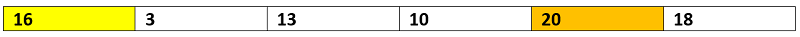So root is 16(highlighted yellow)

Next greater element is 20(highlighted orange)

No element after that 20 is lower than the root value(16)

Now we need to test the left subtree and right subtree

Checking the left subtree part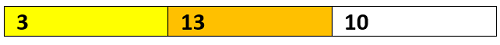So root is 3(highlighted yellow)

Next greater element is 13(highlighted orange)

No element after that 13 is lower than the root value(3)

Now we need to test the left subtree and right subtree for this array

There is no left subtree and it only has the right subtree [13,10]

For [13, 10] there is no next greater element, so it has only left part which is 10.

So the left subtree returns TRUE

Checking the right subtree part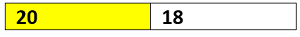So root is 20(highlighted yellow)

There is no Next greater element

So it has no right subtree and the left child will be 18

Thus it again returns TRUE

So overall it returns TRUE and that means it's a valid preorder traversal array for BST

The Binary Search tree whose preorder traversal has been represented here is the below one: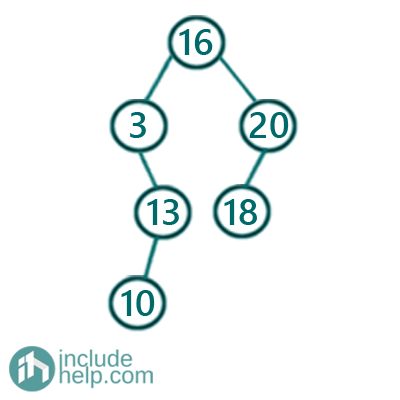Preorder2: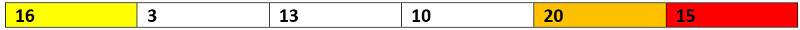So root is 16(highlighted yellow)

Next greater element is 20(highlighted orange)

There is an element(15) after that 20 which is lower than the root value(16)

Thus it's not a valid Binary Search Tree preorder traversal representation.